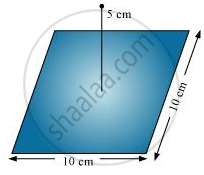Share

# A Point Charge +10 μC is a Distance 5 Cm Directly Above the Centre of a Square of Side 10cm, as Shown in Fig. 1.34. What is the Magnitude of the Electric Flux Through the Square? - CBSE (Science) Class 12 - Physics

#### Question

A point charge +10 μC is a distance 5 cm directly above the centre of a square of side 10 cm, as shown in Fig. 1.34. What is the magnitude of the electric flux through the square? (Hint: Think of the square as one face of a cube with edge 10 cm.)#### Solution

The square can be considered as one face of a cube of edge 10 cm with a centre where charge q is placed. According to Gauss’s theorem for a cube, total electric flux is through all its six faces.

phi_"total"=q/in_0

Hence, electric flux through one face of the cube i.e., through the square, phi=phi_"total"/6

=1/6 q/in_0

Where,

0 = Permittivity of free space

= 8.854 × 10−12 N−1Cm−2

q = 10 μC = 10 × 10−6 C

∴=1/6xx(10xx10^-6)/(8.854xx10^-12)

= 1.88 × 105 N m2 C−1

Therefore, electric flux through the square is 1.88 × 105 N m2 C−1.

Is there an error in this question or solution?

#### APPEARS IN

NCERT Solution for Physics Textbook for Class 12 (2018 to Current)
Chapter 1: Electric Charge and Fields
Q: 18 | Page no. 47

#### Video TutorialsVIEW ALL 

Solution A Point Charge +10 μC is a Distance 5 Cm Directly Above the Centre of a Square of Side 10cm, as Shown in Fig. 1.34. What is the Magnitude of the Electric Flux Through the Square? Concept: Electric Flux.
S